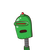# Find the value of p, If the equation3x^2+2x+p=0 and 6x^2-17x+12=0Explain why we get double answer.​

Find the value of p, If the equation
3x^2+2x+p=0 and 6x^2-17x+12=0
Explain why we get double answer.​

### 1 thought on “Find the value of p, If the equation<br />3x^2+2x+p=0 and 6x^2-17x+12=0<br />Explain why we get double answer.​”

1.Step-by-step explanation:

6x²-17x+12=0

=6x²-9x-8x+12=0

=3x(2x-3) -4(2x-3) =0

=(3x-4) (2x-3) =0

• when 3x-4=0

3x=4

x=4/3

• when 2x-3=0

2x=3

x=3/2

NOW IN Equation 1

• 3x²+2x+p=0

WE HAVE TWO VALUE OF X

SO WE GET TWO DIFFERENT ANSWER AFTER PUTTING THE VALUE